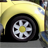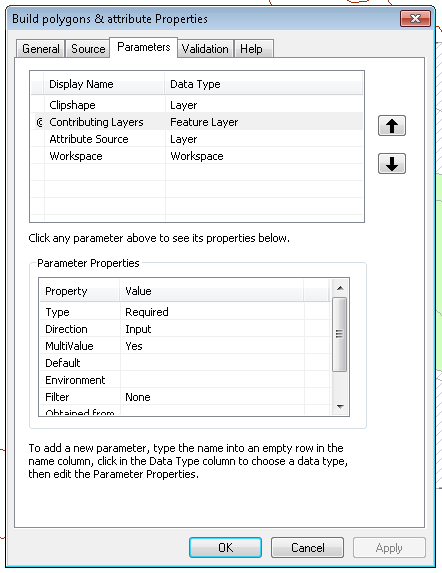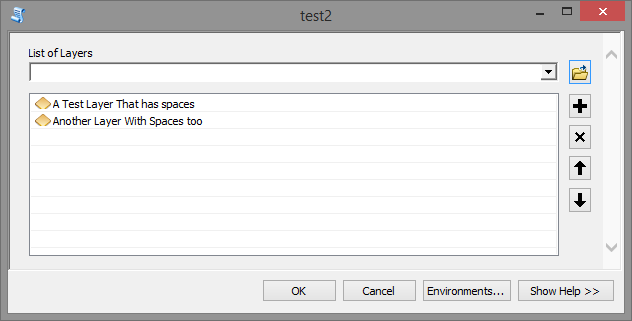# Passing ArcMap layers to a Python script inside ArcToolbox

1988
3
08-29-2016 03:57 AMOccasional Contributor II

Hi

I've got a small script that I've turned into an ArcToolbox tool / script / thingy.

I would like to be able to pass ArcMap layers to the tool (ie drag the layer directly from ToC into the tool, and have the tool run only on the selected features).

By and large, I have this running the way I would expect. However, if I'm trying to pass a layer to the tool that has been renamed in the ToC (ie given a 'proper' name rather than just the feature class name) the tool fails.

For reference, I'm using arcpy.GetParameterAsText to pass variables from the tool to the script, and the particular parameter that is causing issues is a type 'Feature Layer' and is a multivalue parameter.

Does anyone have any crazy suggestions that may fix this issue? I'm out of my depth!

ThanksTags (5)
1 Solution

Accepted SolutionsbyEsri Esteemed Contributor

I don't think it has much to do with the spaces in the names, but more that when you get the list of layer names as a string, you have to turn it into a list and each element will be a string (or unicode). You can avoid this by using the GetParameter (not as text) and use that instead:

Example code this does not run correctly:

``````def main():
import arcpy
list_txt = arcpy.GetParameterAsText(0)
lst = list_txt.split(';')

for lyr in lst:
count = getCount(lyr)

def getCount(fc):
return int(arcpy.GetCount_management(fc).getOutput(0))

if __name__ == '__main__':
main()‍‍‍‍‍‍‍‍‍‍‍‍‍‍‍‍‍``````

Using these inputs:Will throw the following error:

Traceback (most recent call last):
File "D:\Xander\GeoNet\LayerNames\layer_names2.py", line 28, in <module>
main()
File "D:\Xander\GeoNet\LayerNames\layer_names2.py", line 19, in main
count = getCount(lyr)
File "D:\Xander\GeoNet\LayerNames\layer_names2.py", line 24, in getCount
return int(arcpy.GetCount_management(fc).getOutput(0))
File "c:\program files (x86)\arcgis\desktop10.4\arcpy\arcpy\management.py", line 15779, in GetCount
raise e
ExecuteError: Failed to execute. Parameters are not valid.
ERROR 000732: Input Rows: Dataset 'A Test Layer That has spaces' does not exist or is not supported
Failed to execute (GetCount).

But when you change the code to this:

``````def main():
import arcpy
lst = arcpy.GetParameter(0)

for lyr in lst:
count = getCount(lyr)

def getCount(fc):
return int(arcpy.GetCount_management(fc).getOutput(0))

if __name__ == '__main__':
main()
‍‍‍‍‍‍‍‍‍‍‍‍‍‍‍‍``````

It will result in this:3 RepliesOccasional Contributor II

More digging has unearthed the issue, but not the solution -

• If the layer name has a space in it (within the multivalue parameter) the tool will fail.
• If the layer name has the space removed, the tool will complete.
• However, if not using the multivalue option, the parameter will accept a layer, regardless of whether there is a space in the name or not.byEsri Esteemed Contributor

I don't think it has much to do with the spaces in the names, but more that when you get the list of layer names as a string, you have to turn it into a list and each element will be a string (or unicode). You can avoid this by using the GetParameter (not as text) and use that instead:

Example code this does not run correctly:

``````def main():
import arcpy
list_txt = arcpy.GetParameterAsText(0)
lst = list_txt.split(';')

for lyr in lst:
count = getCount(lyr)

def getCount(fc):
return int(arcpy.GetCount_management(fc).getOutput(0))

if __name__ == '__main__':
main()‍‍‍‍‍‍‍‍‍‍‍‍‍‍‍‍‍``````

Using these inputs:Will throw the following error:

Traceback (most recent call last):
File "D:\Xander\GeoNet\LayerNames\layer_names2.py", line 28, in <module>
main()
File "D:\Xander\GeoNet\LayerNames\layer_names2.py", line 19, in main
count = getCount(lyr)
File "D:\Xander\GeoNet\LayerNames\layer_names2.py", line 24, in getCount
return int(arcpy.GetCount_management(fc).getOutput(0))
File "c:\program files (x86)\arcgis\desktop10.4\arcpy\arcpy\management.py", line 15779, in GetCount
raise e
ExecuteError: Failed to execute. Parameters are not valid.
ERROR 000732: Input Rows: Dataset 'A Test Layer That has spaces' does not exist or is not supported
Failed to execute (GetCount).

But when you change the code to this:

``````def main():
import arcpy
lst = arcpy.GetParameter(0)

for lyr in lst:
count = getCount(lyr)

def getCount(fc):
return int(arcpy.GetCount_management(fc).getOutput(0))

if __name__ == '__main__':
main()
‍‍‍‍‍‍‍‍‍‍‍‍‍‍‍‍``````

It will result in this:Occasional Contributor II

Thank you very much Xander. This has fixed the issue!